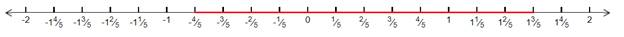# the absolute value inequality. Express the solution using interval notation and graph the solution set.### Precalculus: Mathematics for Calcu...

6th Edition
Stewart + 5 others
Publisher: Cengage Learning
ISBN: 9780840068071### Precalculus: Mathematics for Calcu...

6th Edition
Stewart + 5 others
Publisher: Cengage Learning
ISBN: 9780840068071

#### Solutions

Chapter 1.7, Problem 80E
To determine

## To solve: the absolute value inequality. Express the solution using interval notation and graph the solution set.

Expert Solution

x(45,85) .

### Explanation of Solution

Given:

The given inequality is |5x2|<6 .

Concept used:

Properties of absolute value inequalities:

 Inequality Equivalent form Graph |x|c x<−c or c

Calculation:

According to the properties of absolute value inequalities, the given inequalityis expressed as

6<|5x2|<66+2<|5x2+2|<6+2{add 2 on both the sides}4<|5x|<8{divide 5 on both the sides}45<|5x|<85i.ex(45,85) .

Hence,the solution set is x(45,85) .

The solution set of the inequality graphed on the number line.### Have a homework question?

Subscribe to bartleby learn! Ask subject matter experts 30 homework questions each month. Plus, you’ll have access to millions of step-by-step textbook answers!